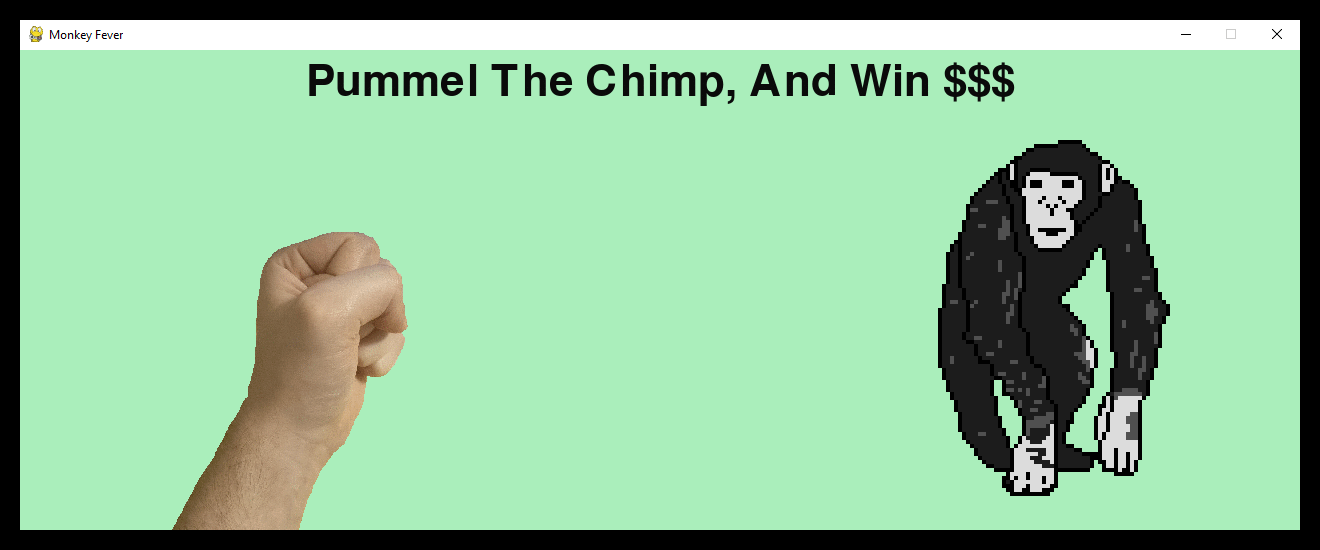## 逐行钳位器¶

pete@shinners.org

### 引言¶

(不，这不是横幅广告，这是截图)Full Source

### 导入模块¶

```# Import Modules
import os
import pygame as pg

if not pg.font:
print("Warning, fonts disabled")
if not pg.mixer:
print("Warning, sound disabled")

main_dir = os.path.split(os.path.abspath(__file__))
data_dir = os.path.join(main_dir, "data")
```

### 正在加载资源¶

```def load_image(name, colorkey=None, scale=1):
fullname = os.path.join(data_dir, name)

size = image.get_size()
size = (size * scale, size * scale)
image = pg.transform.scale(image, size)

image = image.convert()
if colorkey is not None:
if colorkey == -1:
colorkey = image.get_at((0, 0))
image.set_colorkey(colorkey, pg.RLEACCEL)
return image, image.get_rect()
```

```def load_sound(name):
class NoneSound:
def play(self):
pass

if not pg.mixer or not pg.mixer.get_init():
return NoneSound()

fullname = os.path.join(data_dir, name)
sound = pg.mixer.Sound(fullname)

return sound
```

### 游戏对象类¶

```class Fist(pg.sprite.Sprite):
"""moves a clenched fist on the screen, following the mouse"""

def __init__(self):
pg.sprite.Sprite.__init__(self)  # call Sprite initializer
self.fist_offset = (-235, -80)
self.punching = False

def update(self):
"""move the fist based on the mouse position"""
pos = pg.mouse.get_pos()
self.rect.topleft = pos
self.rect.move_ip(self.fist_offset)
if self.punching:
self.rect.move_ip(15, 25)

def punch(self, target):
"""returns true if the fist collides with the target"""
if not self.punching:
self.punching = True
hitbox = self.rect.inflate(-5, -5)
return hitbox.colliderect(target.rect)

def unpunch(self):
"""called to pull the fist back"""
self.punching = False
```

```class Chimp(pg.sprite.Sprite):
"""moves a monkey critter across the screen. it can spin the
monkey when it is punched."""

def __init__(self):
pg.sprite.Sprite.__init__(self)  # call Sprite intializer
self.image, self.rect = load_image("chimp.png", -1, 4)
screen = pg.display.get_surface()
self.area = screen.get_rect()
self.rect.topleft = 10, 90
self.move = 18
self.dizzy = False

def update(self):
"""walk or spin, depending on the monkeys state"""
if self.dizzy:
self._spin()
else:
self._walk()

def _walk(self):
"""move the monkey across the screen, and turn at the ends"""
newpos = self.rect.move((self.move, 0))
if not self.area.contains(newpos):
if self.rect.left < self.area.left or self.rect.right > self.area.right:
self.move = -self.move
newpos = self.rect.move((self.move, 0))
self.image = pg.transform.flip(self.image, True, False)
self.rect = newpos

def _spin(self):
"""spin the monkey image"""
center = self.rect.center
self.dizzy = self.dizzy + 12
if self.dizzy >= 360:
self.dizzy = False
self.image = self.original
else:
rotate = pg.transform.rotate
self.image = rotate(self.original, self.dizzy)
self.rect = self.image.get_rect(center=center)

def punched(self):
"""this will cause the monkey to start spinning"""
if not self.dizzy:
self.dizzy = True
self.original = self.image
```

### 初始化所有内容¶

```pg.init()
screen = pg.display.set_mode((1280, 480), pg.SCALED)
pg.display.set_caption("Monkey Fever")
pg.mouse.set_visible(False)
```

### 创建背景¶

```background = pg.Surface(screen.get_size())
background = background.convert()
background.fill((170, 238, 187))
```

### 将文本置于背景，居中¶

```if pg.font:
font = pg.font.Font(None, 64)
text = font.render("Pummel The Chimp, And Win \$\$\$", True, (10, 10, 10))
textpos = text.get_rect(centerx=background.get_width() / 2, y=10)
background.blit(text, textpos)
```

### 安装完成时显示背景¶

```screen.blit(background, (0, 0))
pygame.display.flip()
```

### 准备游戏对象¶

```whiff_sound = load_sound("whiff.wav")
chimp = Chimp()
fist = Fist()
allsprites = pg.sprite.RenderPlain((chimp, fist))
clock = pg.time.Clock()
```

### 主环路¶

```going = True
while going:
clock.tick(60)
```

### 处理所有输入事件¶

```for event in pg.event.get():
if event.type == pg.QUIT:
going = False
elif event.type == pg.KEYDOWN and event.key == pg.K_ESCAPE:
going = False
elif event.type == pg.MOUSEBUTTONDOWN:
if fist.punch(chimp):
punch_sound.play()  # punch
chimp.punched()
else:
whiff_sound.play()  # miss
elif event.type == pg.MOUSEBUTTONUP:
fist.unpunch()
```

### 更新雪碧¶

```allsprites.update()
```

### 绘制整个场景¶

```screen.blit(background, (0, 0))
allsprites.draw(screen)
pygame.display.flip()
```

### 游戏结束¶

```pg.quit()
```

Edit on GitHub